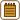## Changing the Melting Point

### Task number: 2178

Molar entropy change of melting ice is 22.2 J mol−1K−1. Determine the change in the melting point if the external pressure has increased from 100 kPa to 200 kPa.

• #### Hint 1

To calculate the temperature change ΔT, use the so-called Clapeyron equation. Determine which quantities in the equation we know and which ones we have to express.

• #### Hint 2

Since the temperature lies in a relatively small range, the molar entropy change ΔSmol, and hence the whole right side of the Clapeyron equation, can be considered constant.

• #### Notation

 ΔSmol = 22.2 J mol−1K−1 molar entropy change of melting ice p1 = 100 kPa = 100 000 Pa initial pressure p2 = 200 kPa = 200 000 Pa final pressure ΔT = ? change in melting point

From the Tables:

 ρw = 1000 kg m−3 density of water ρi = 917 kg m−3 density of ice Mm = 0.018 kg mol−1 molar mass of water
• #### Analysis

The phase transition point depends on pressure. Typical example is a pressure cooker, in which water is boiling at a temperature considerably higher than 100 °C. On the contrary, in high mountains, the boiling point of water is decreased because of the lower pressure.

These changes can be expressed quantitatively using the so-called Clapeyron equation. Basically, it is a differential equation stating that the ratio of the pressure change and phase transition temperature change is equal to the ratio of the molar heat of the transition and the product of the thermodynamic temperature and the difference between the molar volume of the substance in the 2nd and in the 1st phase.

In order to obtain a formula consisting of the quantities given in the task only, we need to adjust the equation. First, we can substitute the ratio of the molar heat and the temperature by the molar entropy change. Second, we express the molar volume, both in the 1st and in the 2nd phase, as the ratio of the molar mass and corresponding density. After we express the pressure change as the difference between the final and initial pressure, we obtain a relation from which we just need to express the temperature change.

• #### Solution

Our calculation is going to be based on the so-called Clapeyron equation, whose most common form is as follows

$\frac{\mathrm{d}p}{\mathrm{d}T}=\frac{L_{mol}}{T(V_{2m}-V_{1m})},$

where p stands for pressure, T for thermodynamic temperature at which the phase transition occurs, Lmol for molar heat and V1m and V2m for molar volume of the substance in the 1st and 2nd phase respectively.

In the formula, molar heat Lmol is unknown. However, remembering that the following relation applies to the molar entropy change ΔSmol

$\mathrm{\Delta}S_{mol}=\frac{L_{mol}}{T},$

we can adjust the original formula as follows

$\frac{\mathrm{d}p}{\mathrm{d}T}=\frac{\mathrm{\Delta}S_{mol}}{V_{2m}- V_{1m}}.$

Then, we also need to express the molar volume of the ice V1m and of the water V2m using the given quantities. We use the known relation

$V_{1m}=\frac{M_{m}}{\rho_{i}},$ $V_{2m}=\frac{M_{m}}{\rho_{w}},$

where Mm is the molar mass of water (as well as ice, of course), ρi density of ice and ρw density of water.

Substituting the expressed quantities in the Clapeyron equation, we obtain

$\frac{\mathrm{d}p}{\mathrm{d}T}=\frac{\mathrm{\Delta}S_{mol}}{\frac{M_{m}}{\rho_{w}}-{\frac{M_{m}}{\rho_{i}}}}.$

Since the temperature lies in a relatively small range, the molar entropy change ΔSmol, and hence the whole right side of the Clapeyron equation, can be considered constant. Thanks to that, we do not have to solve the differential equation and we can just substitute the differential increments for classic “delta”:

$\frac{\mathrm{\Delta}p}{\mathrm{\Delta}T}=\frac{\mathrm{\Delta}S_{mol}}{\frac{M_{m}}{\rho_{w}}-{\frac{M_{m}}{\rho_{i}}}}.$

Therefrom, we can express the required temperature change ΔT right away:

$\mathrm{\Delta}T=\frac{\mathrm{\Delta}p\left(\frac{M_{m}}{\rho_{v}}-{\frac{M_{m}}{\rho_{l}}}\right)}{\mathrm{\Delta}S_{mol}}.$

Finally, we express the pressure change Δp as the difference between the final pressure p2 and the initial one p1

$\mathrm{\Delta}T=\frac {(p_{2}-p_{1}) \left(\frac{M_{m}}{\rho_{w}}-{\frac{M_{m}}{\rho_{i}} }\right)}{\mathrm{\Delta}S_{mol}}.$
• #### Numerical Substitution

$\mathrm{\Delta}T=\frac {(p_{2}-p_{1}) \left(\frac{M_{m}}{\rho_{w}}-{\frac{M_{m}}{\rho_{i}} }\right)}{\mathrm{\Delta}S_{mol}}=\frac {(200\,000-100\,000)\cdot \left(\frac{0.018}{1000}-\frac{0.018}{917}\right)}{22.2}\,\mathrm{K}$ $\mathrm{\Delta}T\dot{=}-0.0073\,\mathrm{K}$
• #### Answer

The melting point of ice decreased by approximately 0.0073 K.

• #### Comment

When using the Clapeyron equation, we need to pay extra attention to the sign.

In our case, water has a higher density than ice, so its molar volume is smaller (V2m < V1m). Thus, if the pressure increases (dp > 0), the phase transition point will logically decrease (dT < 0).

Similar considerations must be done for every task of this type, otherwise the result will be numerically correct, but physically completely absurd!